Select Page

# Cost Volume Profit Analysis Problems and Solutions

Cost Volume Profit Analysis Problems PDF Download

## Problem # 1:

Assume that as an investor, you are planning to enter the construction industry as a panel formwork supplier. The potential number of forthcoming projects, you forecasted that within two years, your fixed cost for producing formworks is Rs. 300,000. The variable unit cost for making one panel is Rs.  15.  The sale price for each panel will be Rs. 25. If you charge Rs. 25 for each panel, how many panels you need to sell in total, in order to start making money?

### Solution: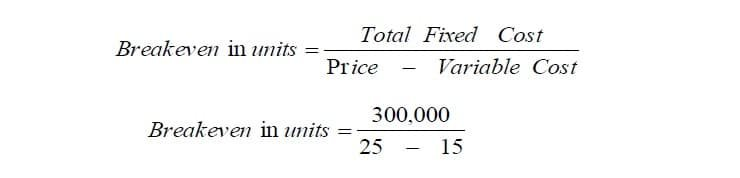Answer: Break-Even in Units = 30,000 panels

>> Practice Cost Volume Profit Analysis MCQs

## Problem # 2:

Suppose you intend to open a franchise business to supply a nationally-known line of women’s shoes.  You’ve found a good location in Abbottabad to open your shop, and have determined that the average prices and costs of operating the store are:

Price = Rs. 50 per pair                                             Cost = Rs. 30 per pair Rent = Rs. 2,500 per month                                     Insurance = Rs. 500 per month Utilities & Telephone = Rs. 300 per month

In addition, you plan to hire two sales ladies on a commission basis of 10% in order to provide them with incentive to sell shoes.  You are required determine the breakeven point in Rupees?

### Solution: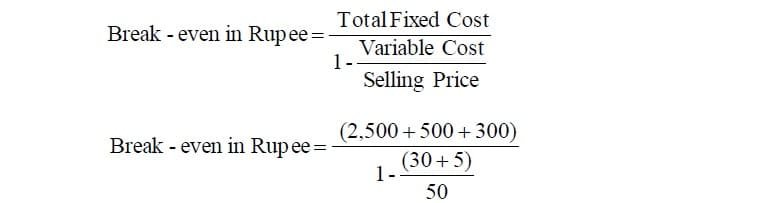Answer: Break-Even in Rupees = Rs. 11,000

>> Readings Cost Volume Profit Analysis.

## Problem # 3:

A manufacturing company supplies its products to construction job sites. The average monthly fixed cost per site is Rs. 4,500, while each unit cost Rs. 35 to produce and selling price is Rs. 50 per unit. Determine the monthly breakeven volume.

### Solution: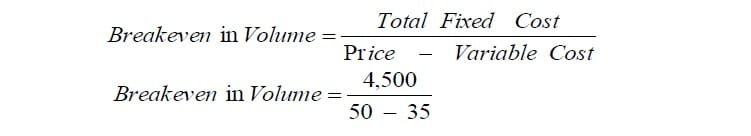Answer: Break-Even in Volume = 300

>> Further Readings Process Costing.

## Problem # 4:

A store sells t-shirts.  The average selling price is Rs. 15 and the average variable cost (cost price) is Rs. 9.  Thus, every time the store sells a shirt it has Rs. 6 remaining after it pays the manufacturer.  This Rs. 6 is referred to as the unit contribution.

(a) Suppose the fixed costs of operating the store (its operating expenses) are Rs. 100,000 per year.  Find Break-even in units?

### Solution: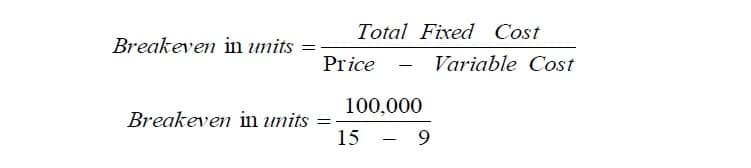Answer: Break-Even in Units = 16,667 T-shirts

(b) If the owner desired a profit of Rs. 25,000, what will be break-even point in Rupees?

Solution:

###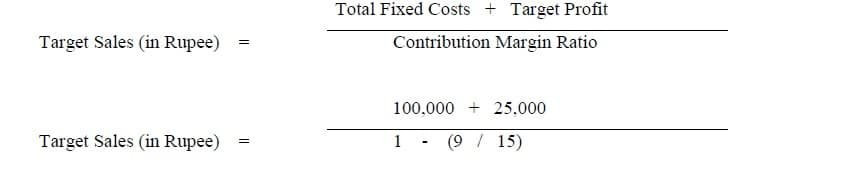Answer: Break-Even in Rupees = 16,667

(c) If fixed costs rose to Rs. 110,000, break-even in units volume would be?   Solution: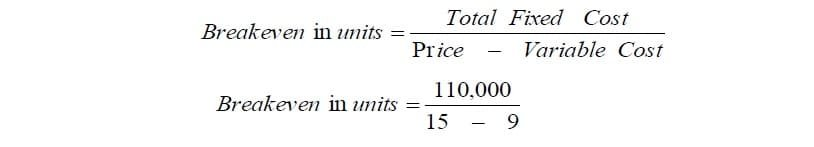Answer: Break-Even in Units = 18,333 T-shirts

(d) If the average selling price rose to Rs.16, break even volume would fall?   Solution: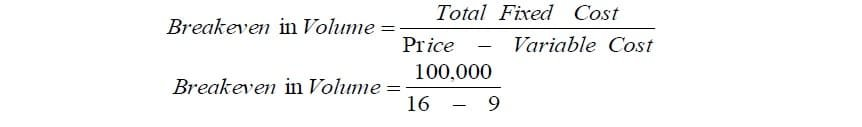Answer: Break-Even in Volume = 14,286 T-shirts

>> Further Readings Job Order Costing.

## Related Topics

Cost Volume Profit Analysis PDF

Cost Volume Profit Analysis

## Related Courses

Principles of Accounting

Cost Accounting

Principles of Finance

1.Thanks you very much

2.Thanks you for your’s help!!

3.Gray ltd wish to sell 10,000 units of its product which has a variable cost of \$20. Fixed cost is \$46,000\$ and required profit is 24,000\$ . Calculate selling price per unit

•Fixed Cost (46,000) + Net Operating Income (24,000)= Contribution Margin (70,000)
Variable Cost per unit (20) * Sale Units (10,000) = Variable cost (200,000)
Contribution Margin (70,000) + Variable Cost (200,000) = Selling Price (270,000)

Either
Contribution Margin(70,000) / Unit of Sales (10,000)= CM units = 7
THUS Variable Cost per unit (20) + CM units (7) = Selling price per unit (27)

4.Break Even Analysis in economics, financial modeling, and cost accounting refers to the point in which total cost and total revenue are equal. It is used to determine the number of units or revenue needed to cover total costs (fixed